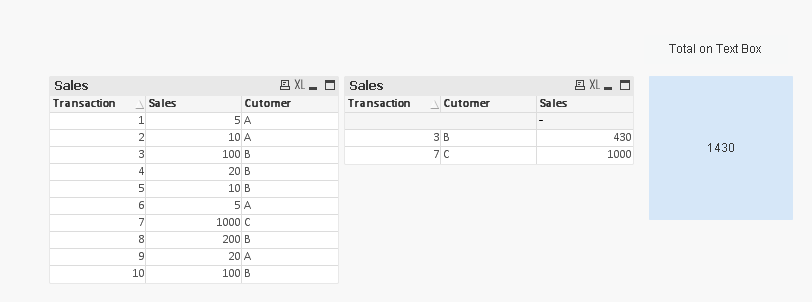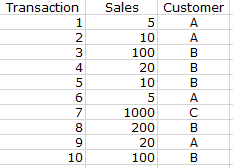# QlikView App Development

Discussion Board for collaboration related to QlikView App Development.

HighlightedNot applicable

## Sum of an aggregation if the aggregation meets a calculated threshold

Hi Community,

I am trying to get the sum sales of all customers that have total sales above a certain level. The issue I'm running into is that the data is at a transactional level, so the formula must first aggregate sales at the customer level and then assess those aggregated values.

I have tried the following without luck:

IF(

AGGR(SUM(Sales), Customer) >= v.CUSTFRACTILE,

SUM(SALES))

and

IF(

AGGR(SUM(Sales), Customer) >= v.CUSTFRACTILE,

SUM(AGGR(SUM(Sales), Customer)))

Any help would be greatly appreciated!

Thank you,

Tags (1)
1 Solution

Accepted Solutions
New Contributor III

## Re: Sum of an aggregation if the aggregation meets a calculated threshold

Something like this ?Regards!

9 Replies
Esteemed Contributor III

## Re: Sum of an aggregation if the aggregation meets a calculated threshold

Can you share some sample data with an expected output.Not applicable

## Re: Sum of an aggregation if the aggregation meets a calculated threshold

Hi Vishwarath,

I can't share the actual data but I have created a simple example below:If in this example I'm looking for the sum of sales for all customers that have sales of at least 400, the correct answer would be 1430 (sum of sales for customers B and C since they spent 430 and 1000 respectively).

Esteemed Contributor III

## Re: Sum of an aggregation if the aggregation meets a calculated threshold

Are you looking for this?Assuming that you already have set the variable vCustfractile to 400.

Then in your straight table add Customer and Transaction and expression as

= IF(Sum(Aggr(Sum(SalesAmt), Customer)) >= vCustfractile, Sum(Aggr(Sum(SalesAmt), Customer)))

With Total Mode as Sum of Rows Radio check.Not applicable

## Re: Sum of an aggregation if the aggregation meets a calculated threshold

Thanks, Vishwarath.

I'm looking to get the number to display in a text  box since I'm using it as a KPI at the top of a dashboard. Is there a way of calculating this without using a straight table (or alternately referencing a straight table total from a text box)?

New Contributor III

## Re: Sum of an aggregation if the aggregation meets a calculated threshold

Something like this ?Regards!Not applicable

## Re: Sum of an aggregation if the aggregation meets a calculated threshold

The SUM(IF( statement works perfectly - Thank you!

Contributor II

## Re: Sum of an aggregation if the aggregation meets a calculated threshold

How to get this 1430 in a text box using set expression?  assuming we have vCUSTFRACTILE as variable.MVP

## Re: Sum of an aggregation if the aggregation meets a calculated threshold

May be this

=Sum({<Cutomer = {"=Sum(Sales) > \$(vCUSTFRACTILE)"}>}Sales)

Contributor II

## Re: Sum of an aggregation if the aggregation meets a calculated threshold

Perfect thanks Much Sunny.